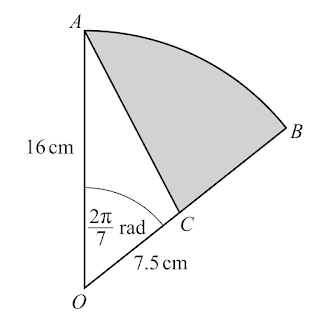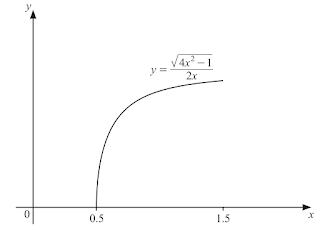### Subscribe Us# CIE0606/2021/m/22 Question

1. Solve the equation $|4 x+9|=|6-5 x|$. 

2 Find the values of the constant $k$ for which the equation $k x^{2}-3(k+1) x+25=0$ has equal roots. $$

3 The diagram shows the graph of $y=\mathrm{f}(x)$, where $\mathrm{f}(x)=a(x+b)^{2}(x+c)$ and $a, b$ and $c$ are integers.

(a) Find the value of each of $a, b$ and $c$.

(b) Hence solve the inequality $\mathrm{f}(x) \leqslant-1$ $$

4 The curve $\dfrac{4}{x^{2}}+\dfrac{5}{4 y^{2}}=1 \quad$ and the line $\quad x+2 y=0 \quad$ intersect at two points. Find the exact distance between these points. $\quad$

5 A cube of side $x \mathrm{~cm}$ has surface area $S \mathrm{~cm}^{2}$. The volume, $V \mathrm{~cm}^{3}$, of the cube is increasing at a rate of $480 \mathrm{~cm}^{3} \mathrm{~s}^{-1}$. Find, at the instant when $V=512$,

(a) the rate of increase of $x$,

(b) the rate of increase of $S$.6 $A O B$ is a sector of a circle with centre $O$ and radius $16 \mathrm{~cm}$. Angle $A O B$ is $\dfrac{2 \pi}{7}$ radians. The point $C$ lies on $O B$ such that $O C$ is of length $7.5 \mathrm{~cm}$ and $A C$ is a straight line.

(a) Find the perimeter of the shaded region.

(b) Find the area of the shaded region. $$

7. A curve has equation $y=p(x)$, where $p(x)=x^{3}-4 x^{2}+6 x-1$

(a) Find the equation of the tangent to the curve at the point $(3,8)$. Give your answer in the form $y=m x+c . \quad$

(b) (i) Given that $\mathrm{p}^{-1}$ exists, write down the gradient of the tangent to the curve $y=\mathrm{p}^{-1}(x)$ at the point $(8,3) .$

(ii) Find the coordinates of the point of intersection of these two tangents.

8 A photographer takes 12 different photographs. There are 3 of sunsets, 4 of oceans, and 5 of mountains.

(a) The photographs are arranged in a line on a wall.

(i) How many possible arrangements are there if there are no restrictions? $$

(ii) How many possible arrangements are there if the first photograph is of a sunset and the last photograph is of an ocean? $\quad$

(iii) How many possible arrangements are there if all the photographs of mountains are next to each other?

(b) Three of the photographs are to be selected for a competition.

(i) Find the number of different possible selections if no photograph of a sunset is chosen. $\quad$

(ii) Find the number of different possible selections if one photograph of each type (sunset, ocean, mountain) is chosen.

9 (a) In the expansion of $\left(2 k-\dfrac{x}{k}\right)^{5}$, where $k$ is a constant, the coefficient of $x^{2}$ is 160 . Find the value of $k$. $$

(b) (i) Find, in ascending powers of $x$, the first 3 terms in the expansion of $(1+3 x)^{6}$, simplifying the coefficient of each term.

(ii) When $(1+3 x)^{6}(a+x)^{2}$ is written in ascending powers of $x$, the first three terms are $4+68 x+b x^{2}$, where $a$ and $b$ are constants. Find the value of $a$ and of $b .$

10 The function $\mathrm{f}$ is defined by $\mathrm{f}(x)=\dfrac{\sqrt{4 x^{2}-1}}{2 x}$ for $0.5 \leqslant x \leqslant 1.5$.

The diagram shows a sketch of $y=\mathrm{f}(x)$.(a) (i) It is given that $\mathrm{f}^{-1}$ exists. Find the domain and range of $\mathrm{f}^{-1}$.

(ii) Find an expression for $\mathrm{f}^{-1}(x)$.

(b) The function $g$ is defined by $g(x)=\mathrm{e}^{x^{2}}$ for all real $x$. Show that $\operatorname{gf}(x)=\mathrm{e}^{\left(1-\dfrac{a}{b x^{2}}\right)}$, where $a$ and $b$ are integers.

11 $\begin{array}[t]{lll}\mathbf{} & \text { (a) (i) Find } & \displaystyle\int\dfrac{1}{(10 x-1)^{6}} \mathrm{~d} x \text { . }\end{array}$

(ii) Find $\displaystyle\int \dfrac{\left(2 x^{3}+5\right)^{2}}{x} \mathrm{~d} x$.

(b) (i) Differentiate $y=\tan (3 x+1)$ with respect to $x$.

(ii) Hence find $\displaystyle\int_{\dfrac{\pi}{12}}^{\dfrac{\pi}{10}}\left(\dfrac{\sec ^{2}(3 x+1)}{2}-\sin x\right) \mathrm{d} x$.

12 A particle $P$ travels in a straight line so that, $t$ seconds after passing through a fixed point $O$, its velocity, $v \mathrm{~ms}^{-1}$, is given by $v=\dfrac{t}{2 \mathrm{e}}$ for $0 \leqslant t \leqslant 2$, $v=\mathrm{e}^{-\dfrac{t}{2}} \quad$ for $t>2$.

Given that, after leaving $O$, particle $P$ is never at rest, find the distance it travels between $t=1$ and $t=3 .$

****

1 $x=-1/3,x=15$

2 $k=9$ or $1/9$

3a) $a = 2, b = 1, c = −1$

b)  $x\le -1.45$ or $-.4\le 0.85$

4 $3\sqrt 5$

5a) $2.5$

b) 240

6a) 35.6 b) 68.0

7a) $y=9x-19$ bi) 1/9 ii) $(19/8,19/8)$

8a (i)479001600 (ii)43345600  (iii) 4838400

b(i) 84 (ii) 60

9(a) $k=2$

(b)(i) $1+18x+135x^2$

(ii) $a=-2,b=469$

10 (ai) Domain of $f^{-1}:0\le x\le\dfrac{2\sqrt 2}{3}$

Range of $f^{-1}:0.5\le f^{-1}\le 1.5$

(ii) $f^{-1}(x)=\sqrt{\dfrac{1}{4-4x^2}}$

(b) Show

11(a) (i) $\dfrac{(10x-1)^{-5}}{-50}(+c)$

(ii) $\dfrac 46x^6+\dfrac{20}{3}+25\ln x+c$

(b)(i) 3 sec$^2(3x+1)$ (ii) 0.322

12. 0.565